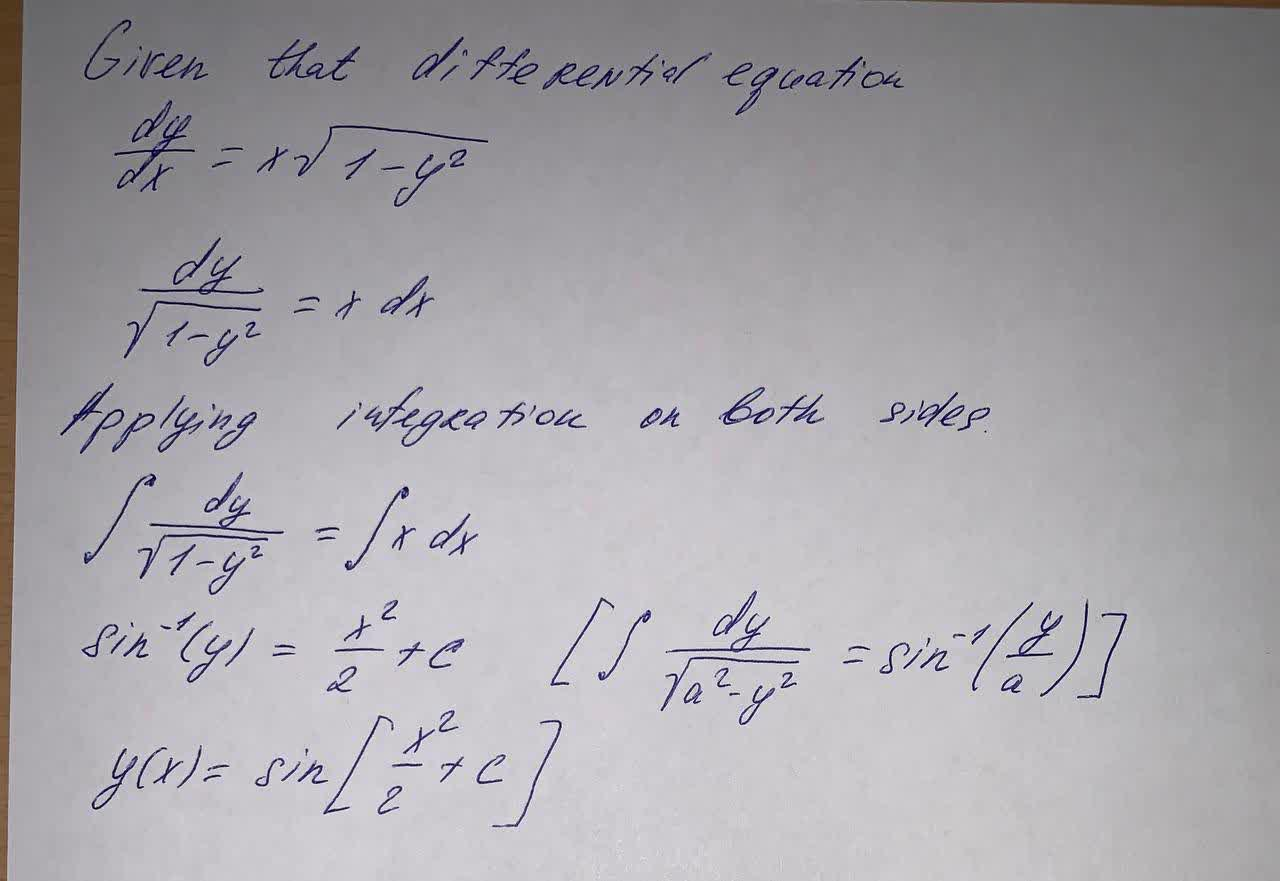Question# Solve the given differential equation by separation of variables: \frac{dy}{dx}=x\sqrt{1-y^2}

Differential equations
ANSWEREDSolve the given differential equation by separation of variables: $$\displaystyle{\frac{{{\left.{d}{y}\right.}}}{{{\left.{d}{x}\right.}}}}={x}\sqrt{{{1}-{y}^{{2}}}}$$